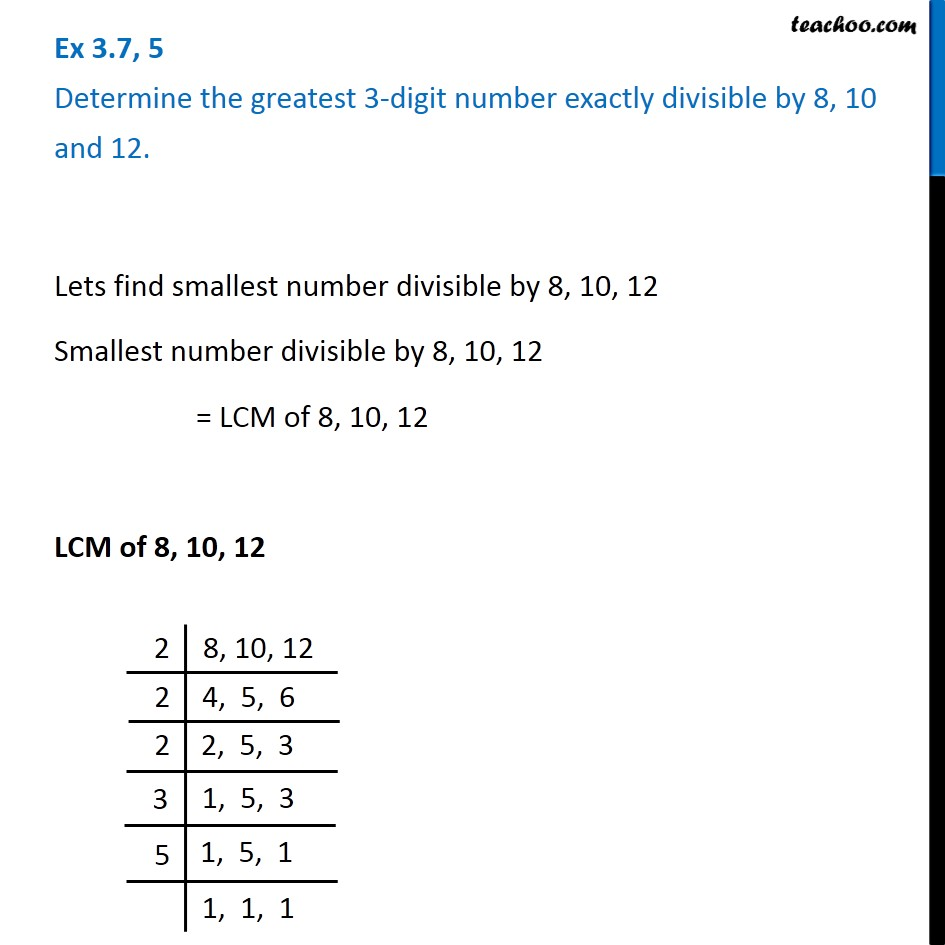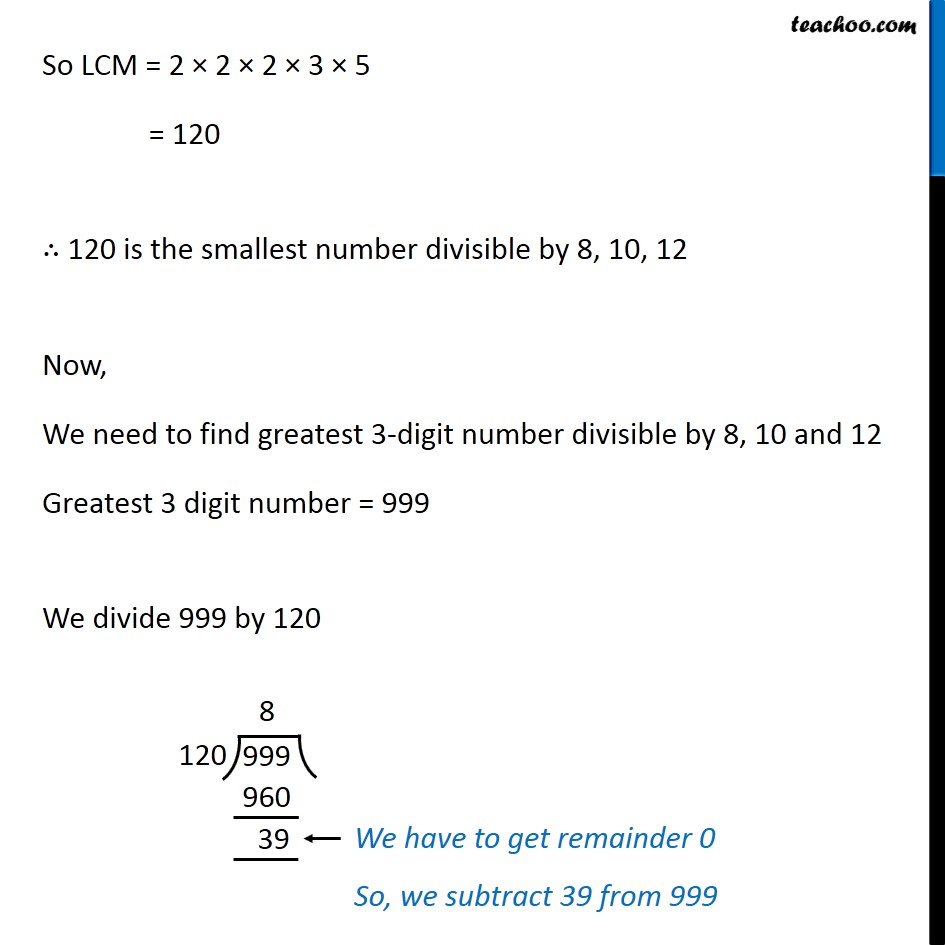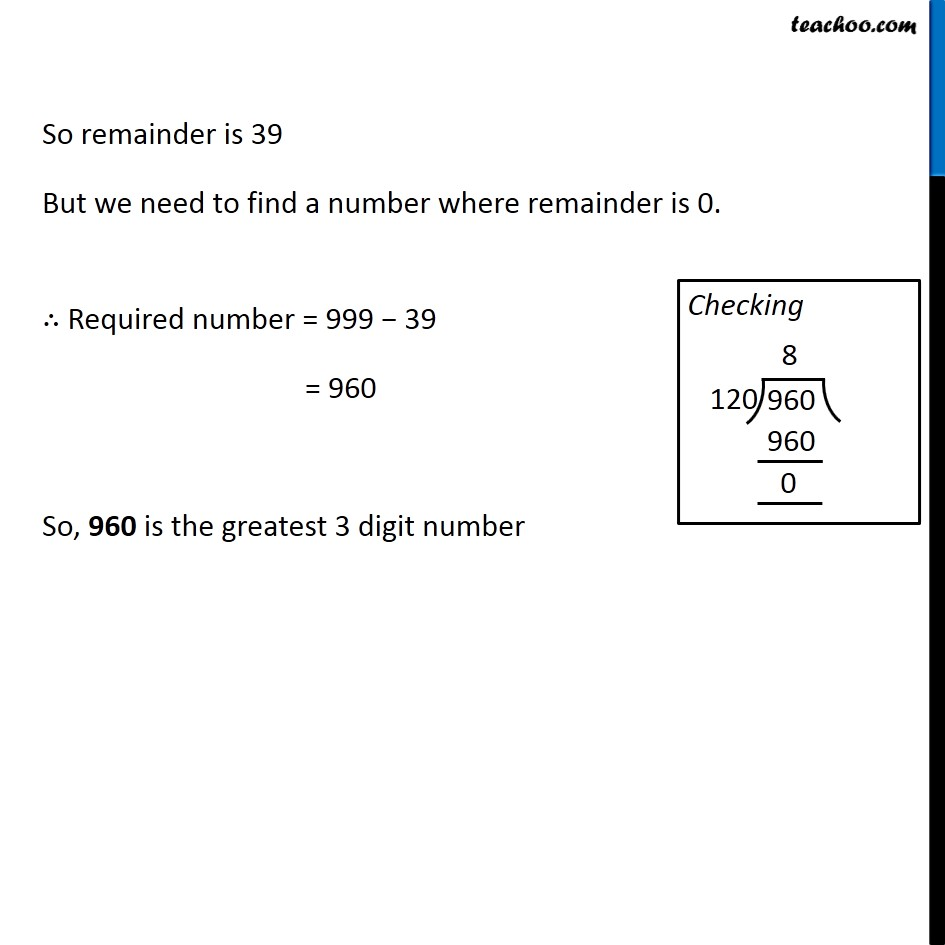Ex 3.7

Chapter 3 Class 6 Playing with Numbers
Serial order wiseLearn in your speed, with individual attention - Teachoo Maths 1-on-1 Class

### Transcript

Lets find smallest number divisible by 8, 10, 12 Smallest number divisible by 8, 10, 12 = LCM of 8, 10, 12 LCM of 8, 10, 12 So LCM = 2 × 2 × 2 × 3 × 5 = 120 ∴ 120 is the smallest number divisible by 8, 10, 12 Now, We need to find greatest 3-digit number divisible by 8, 10 and 12 Greatest 3 digit number = 999 We divide 999 by 120 So remainder is 39 But we need to find a number where remainder is 0. ∴ Required number = 999 − 39 = 960 So, 960 is the greatest 3 digit number